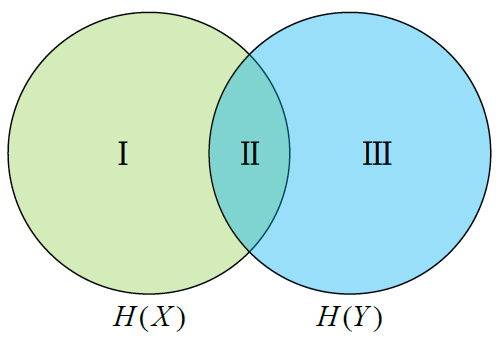# 第一章 信息论的基本概念--鉴别信息【含答案】 应用信息论基础

（1）单选题A  H(X | Y), H(X, Y), H(Y | X)

B  H(Y | X), H(X, Y), H(X | Y)

C  H(X | Y), I(X; Y), H(Y | X)

D  H(Y | X), I(X; Y), H(X | Y)

（2）多选题

A  可以取正值

B  可以为零

C  可以取负值

D  可以等于H(X)

（3）单选题

A  非负性 D(p || q) >= 0

B  对称性 D(p || q) = D(q || p)

C  三角不等式 D(p || r) <= D(p || q) + D(q || r)